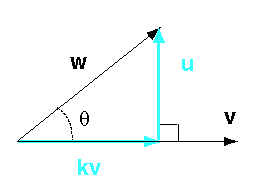w projected onto v is NOT the same as v projected onto w

The vector resulting from a projection is oriented in the direction of the vector projected onto.

# Finding uAfter you have found kv it is easy to find the orthogonal vector, u:

w = kv + u,   so   u = w - kv

In the above formula, "+" means vector addition and "-" means vector subtraction.

So far in this chapter the vectors have all been geometrical vectors. There has been no mention of coordinate frames or column matrices.

### QUESTION 7:

Will these results work when vectors are represented with column matrices?